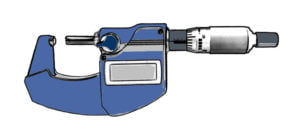# What is the Least Count of Micrometer?

Micrometer’s least count means the smallest increment figure that the micrometer’s scale provides. It’s also the smallest thickness or length that can be measured.

For example 0, 1, 2, 3, 4, 5, 6, 7, 8, 9, 10, these row of numbers has 1 increment figure. Another example, 0.1, 0.2, 0.3, 0.4, 0.5, 0.6, 0.7, 0.8, 0.9, 1.0 and so forth consists of 0.1 increment.

In terms of rulers or tape measures, the least count can be simply interpreted as the distance between each mark. However, it doesn’t totally behave in the micrometer. Need to remember that the scale on the thimble is the amplification of the scale on the sleeve. Therefore, even though the marks’ distance on the thimble’s scale looks 1mm separated each other, the real value is actually smaller than it is.## How the Least Count of Micrometer Gained?

When it comes to the least count of a micrometer, you need to understand first how a micrometer works. To get a better illustration of what it means, you may go to “How Micrometers Work” article.

In a mechanical micrometer, there is a part called spindle in which the threads are spiced according to the requirement. On the threads, there are pitches which further determines the least count of the micrometer. These pitches are divided into smaller divisions with the help of the second scale.

The least count figure is gained based on the pitch length and the number of divisions on the thimble scale. In some micrometers, the least count is also determined by another scale which is called vernier scale. At this type of scale, the lest count is tinier again. So, it has better precision than the rest of it.

## Least Count Formula

The pitch length and divisions then are calculated to get the least count. This gaining can be illustrated easily by the formula below.

The Least Count = Pitch Length / The Total Graduations on Thimble’s Scale

Pitch length is equal to the distance in the axial direction that the spindle surpasses. It’s divided by graduations (the number of divisions) on thimble’s scale. For example, pitch length is 1 mm and the graduations are 50, then the least count is 0.02mm (1/50=0.02). The smaller the pitch length, the better the least count. Similarly, the bigger the number of graduations, the better the least count.

It takes time to disassemble the micrometer in order to measure the pitch length on the spindle. However, the fastest way is to spin the thimble in fully one rotation and look at how far it moves in the axial direction on the sleeve’s scale. If it moves for 1mm in length, then that’s the pitch length.

## The Least Count of Digital Micrometer

The way of digital micrometers work is different from mechanical ones. It employs the capacitor to measure the distance. So, the least count is determined by the ability of the capacitor sensitivity in terms of measuring displacement.

Unfortunately, you cannot notify the least count through the capacitor. However, it’s easier than you might expect. The total digit on the LCD is the maximum capability of micrometer in displaying the measurement result. So, you just have to look at it.

## What is the Recommended Least Count?

Identifying the micrometer least count is important. It’s one of the factors that you need to know. It also shows the quality of precision of the micrometer can gain. Furthermore, it unsurprisingly determines the range price of micrometers.

The best micrometer should be able to have at least 0.001mm least count. As its name micro and also the conversion from 1 millimeter to micrometer is 1000 (1mm=1000µm), that 0.001mm value is reasonable. However, you can pick out another least count if accuracy is not on demand.